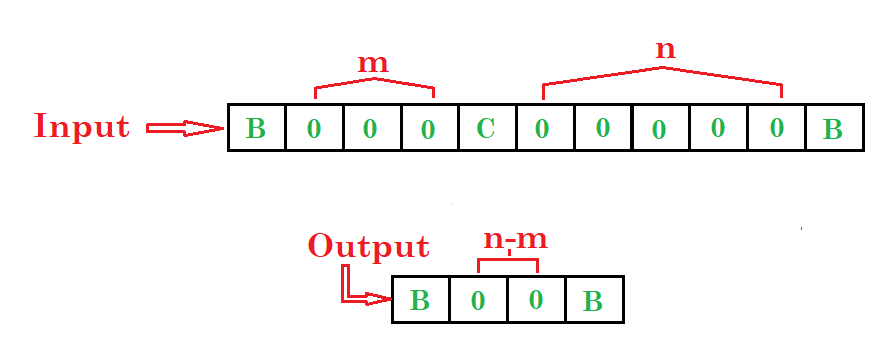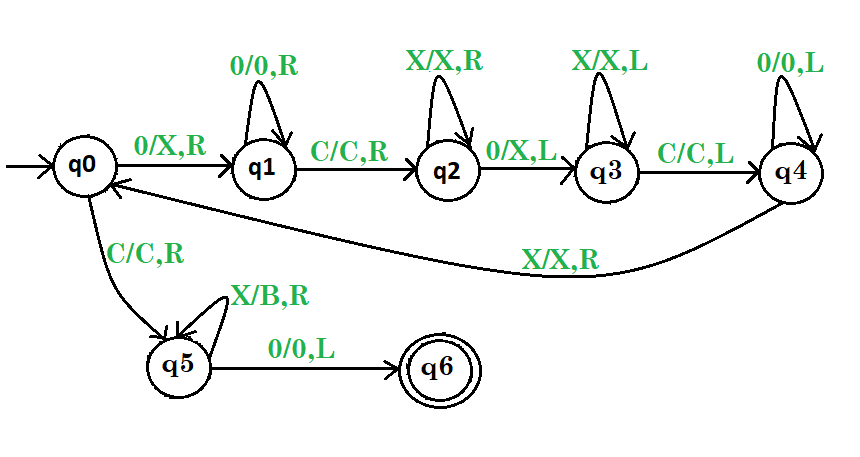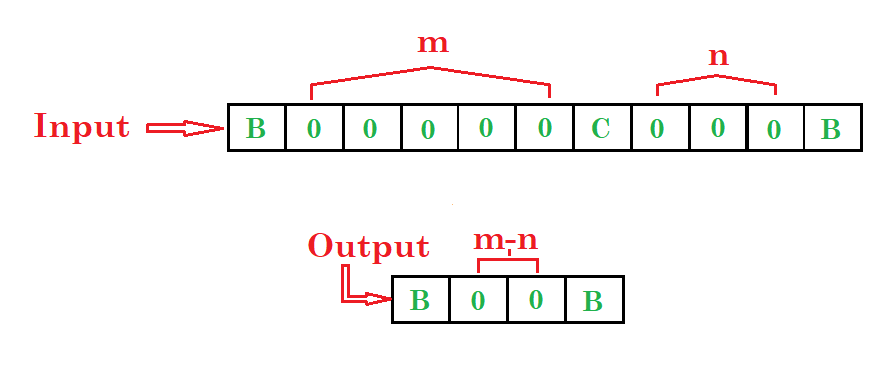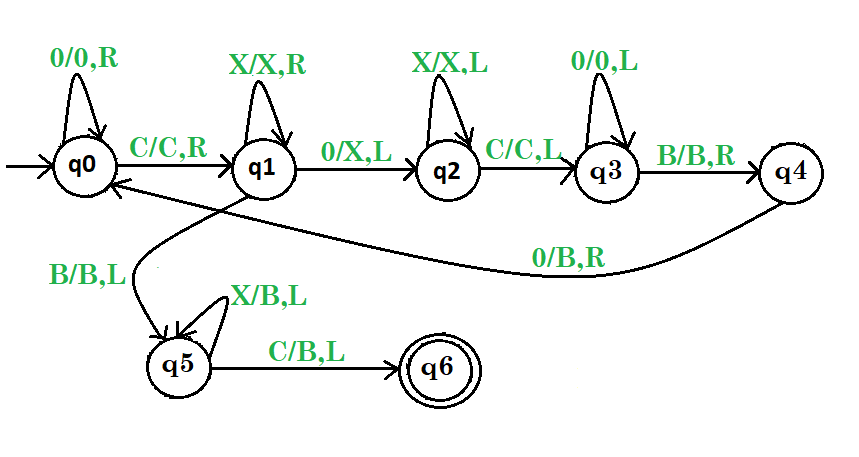GFG App
Open AppBrowser
Continue

# Turing machine for subtraction | Set 1

Prerequisite – Turing Machine Problem-1: Draw a Turing machine which subtract two numbers. Example:Steps:

• Step-1. If 0 found convert 0 into X and go right then convert all 0’s into 0’s and go right.
• Step-2. Then convert C into C and go right then convert all X into X and go right.
• Step-3. Then convert 0 into X and go left then convert all X into X and go left.
• Step-4. Then convert C into C and go left then convert all 0’s into 0’s and go left then convert all X into X and go right and repeat the whole process.
• Step-5. Otherwise if C found convert C into C and go right then convert all X into B and go right then convert 0 into 0 and go left and then stop the machine.Here, q0 shows the initial state and q1, q2, q3, q4, q5are the transition states and q6shows the final state. And X, 0, C are the variables used for subtraction and R, L shows right and left. Problem-2: Draw a Turing machine which subtract two numbers m and n, where m is greater than n.Steps:

• Step-1. If 0 found convert all 0’s into 0’s and go right then convert C into C and go right
• Step-2. If X found then convert all X into X and go right or if 0 found then convert 0 into X and go left and go to next step otherwise go to 5th step
• Step-3. Then convert all X into X and go left then convert C into C and go left
• Step-4. Then convert all 0’s into 0’s and go left then convert B into B and go right then convert 0 into B and go right and repeat the whole process
• Step-5. Otherwise if B found convert B into B and go left then convert all X into B and go left then convert C into B and go left and then stop the machine.Here, q0 shows the initial state and q1, q2, q3, q4, q5are the transition states and q6shows the final state. And B, X, 0, C are the variables used for subtraction(m>n) and R, L shows right and left and B variable is a input symbol. See for – Turing Machine for subtraction | Set 2

My Personal Notes arrow_drop_up